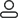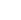LanguageLogin|Sign up
Blog >> Blog Details Page
Ground interference analysis and suppression method in PCB layout
Posted:06:38 PM October 24, 2018 writer: G

1. Ground definition

What is the ground wire? The ground line defined by everyone in textbooks is that the ground line is the equipotential body that serves as the reference point for the circuit potential. This definition is not in line with the actual situation. The potential on the actual ground is not constant. If you use a meter to measure the potential between points on the ground, you will find that the potential at each point on the ground may vary greatly. It is these potential differences that cause the circuit to work abnormally. The definition of a circuit that is an equipotential body is only what people expect from the ground potential.

2. Ground impedance

When talking about the potential difference between the points on the ground caused by the impedance of the ground wire, it can cause the circuit to malfunction. Many people think it is incredible: when we use the ohmmeter to measure the resistance of the ground wire, the resistance of the ground wire is often in the milliohm level. How can such a large voltage drop occur when current flows through such a small resistor, causing abnormal operation of the circuit. To understand this problem, we must first distinguish the two different concepts of resistance and impedance of the wire. The resistance refers to the impedance of the wire to the current in the DC state, and the impedance refers to the impedance of the wire to the current in the AC state. This impedance is mainly caused by the inductance of the wire. Any wire has an inductance. When the frequency is high, the impedance of the wire is much larger than the DC resistance. In the actual circuit, the signal causing electromagnetic interference is often a pulse signal, and the pulse signal contains a rich high-frequency component, so a large voltage is generated on the ground. For digital circuits, the operating frequency of the circuit is very high, so the effect of ground impedance on digital circuits is considerable.

If the impedance at 10 Hz is approximately considered to be a DC resistance, it can be seen that when the frequency reaches 10 MHz, its impedance is 1000 times to 100,000 times that of the DC resistance for a 1 meter long wire. Therefore, for RF current, the voltage drop is large when current flows through the ground. It can also be seen from the table that increasing the diameter of the wire is very effective for reducing the DC resistance, but the effect on reducing the AC impedance is limited. But in electromagnetic compatibility, people are most concerned about the AC impedance. In order to reduce the AC impedance, an effective method is to connect multiple wires in parallel. When two wires are connected in parallel, the total inductance L is:

L = ( L1 + M ) / 2

Where L1 is the inductance of a single wire and M is the mutual inductance between the two wires. It can be seen from the equation that when the two wires are far apart, the mutual inductance between them is small, and the total inductance is equivalent to half of the inductance of a single wire. Therefore, we can reduce the grounding impedance through multiple grounding wires. However, it should be noted that the distance between multiple wires cannot be too close.

3. Ground interference mechanism

3.1 Ground loop interference

Due to the presence of ground impedance, when current flows through the ground, a voltage is generated on the ground. This voltage can be large when the current is large. For example, when a high-power electric appliance is started nearby, a strong current flows through the ground. This current will generate current on the connecting cables of the two devices. Due to the imbalance of the circuit, the current on each wire is different, so differential mode voltage is generated, which affects the circuit. Since this interference is caused by the loop current composed of the cable and the ground, it becomes a ground loop interference. The current in the ground loop can also be induced by the external electromagnetic field.

3.2 Common impedance interference

When two circuits share a ground line, the ground potential of one circuit is modulated by the operating current of the other circuit due to the impedance of the ground line. The signals in such a circuit are coupled into another circuit, and this coupling is referred to as common impedance coupling.

In digital circuits, the ground line tends to exhibit a large impedance due to the high frequency of the signal. At this time, if there are different circuits sharing a ground line, the problem of common impedance coupling may occur.

4. Ground interference countermeasure

4.1 Ground loop countermeasures

From the mechanism of ground loop interference, it can be known that ground loop interference can be reduced by reducing the current in the ground loop. If the current in the ground loop can be completely eliminated, the problem of ground loop interference can be completely solved. Therefore, we propose the following solutions to solve the ground loop interference.

A. Float the device at one end. If one end of the circuit is floating, the ground loop is cut off, so the ground loop current can be eliminated. But there are two issues to be aware of, one is for security reasons, and often does not allow the circuit to float. At this point, consider grounding the device through an inductor. Thus, the grounding impedance of the 50 Hz alternating current device is small, and for the higher frequency interference signal, the grounding impedance of the device is larger, and the ground loop current is reduced. However, this can only reduce the ground loop interference of high frequency interference. Another problem is that although the device is floating, there is still a parasitic capacitance between the device and the ground. This capacitor provides a lower impedance at higher frequencies and therefore does not effectively reduce the high-frequency ground loop current.

B. Use a transformer to connect the devices. Use a magnetic circuit to connect the two devices to cut off the ground loop current. However, it should be noted that the parasitic capacitance between the primary and secondary of the transformer can still provide a path for the higher frequency ground loop current. Therefore, the method of transformer isolation has a poor effect on suppressing the high frequency ground loop current. One way to improve the high-frequency isolation of the transformer is to provide a shield between the primary and secondary of the transformer. However, it must be noted that the ground of the isolation transformer shield must be at the receiving circuit end. Otherwise, not only can the high-frequency isolation effect be improved, but also the high-frequency coupling can be made more serious. Therefore, the transformer is to be mounted on one side of the signal receiving device. A well-shielded transformer provides effective isolation at frequencies below 1 MHz.

C. Using Optical Isolators Another way to cut the ground loop is to use light to achieve signal transmission. This can be said to be the most ideal way to solve the problem of ground loop interference. There are two ways to connect optically, one is an optocoupler device and the other is connected by fiber optics. The optocoupler's parasitic capacitance is typically 2pf, providing good isolation at very high frequencies. Fibers have almost no parasitic capacitance, but they are not as good as optocouplers in terms of installation, maintenance, and cost.

D. Using a common mode choke on a connecting cable using a common mode choke is equivalent to increasing the impedance of the ground loop so that the ground loop current is reduced by a certain ground voltage. But pay attention to control the parasitic capacitance of the common mode choke, otherwise the isolation effect on high frequency interference is very poor. The larger the number of turns of the common mode choke, the larger the parasitic capacitance and the worse the effect of high frequency isolation.

4.2 Elimination of common impedance coupling

There are two ways to eliminate the common impedance coupling. One is to reduce the impedance of the common ground line, so that the voltage on the common ground line is also reduced, thus controlling the common impedance coupling. Another method is to avoid the common grounding of the circuit which is easy to interfere with each other by an appropriate grounding method. Generally, the grounding circuit of the strong electric circuit and the weak electric circuit is avoided, and the digital circuit and the analog circuit share the ground line. As mentioned earlier, the core problem of reducing the ground impedance is to reduce the inductance of the ground. This includes the use of flat conductors for grounding and the use of multiple parallel conductors that are far apart. For printed circuit boards, the ground wire grid on the double-layer board can effectively reduce the ground line impedance. In the multi-layer board, the ground wire is specially used for one layer, although it has a small impedance, but this will increase the cost of the circuit board. . The method of grounding to avoid common impedance by proper grounding is parallel single-point grounding. The disadvantage of parallel grounding is that there are too many grounded conductors. Therefore, in practice, it is not necessary to connect all circuits in parallel at a single point. For circuits with less mutual interference, single-point grounding in series can be used. For example, the circuit can be classified according to strong signals, weak signals, analog signals, digital signals, etc., and then grounded in series with a single point in the same type of circuit, and different types of circuits are connected in parallel with a single point.

634 1 0 1
• PCB
Prototype
• PCB
Assembly
• SMD
Stencil
 Dimensions: (mm) × Quantity: (pcs) 5 5 10 15 20 25 30 40 50 75 100 120 150 200 250 300 350 400 450 500 600 700 800 900 1000 1500 2000 2500 3000 3500 4000 4500 5000 5500 6000 6500 7000 7500 8000 9000 10000 Other Quantities:(quantity*length*width is greater than 10㎡) OK Layers: 1 2 4 6 8 10 12 Thickness: 0.6 mm 0.8 mm 1.0 mm 1.2 mm 1.6 mm 2.0 mm 2.5 mmQuote now